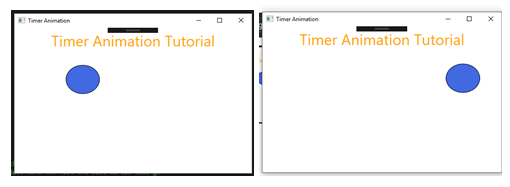# WPF C# Tutorial – Create a Simple Timer Animation in Visual Studio

### Start the C# Programming for Animation

This is the default code which visual studio automatically adds to the code. We will add our own code, so the blue circle can go between right to left of the screen.

Before we can go and start animating everything we need to add another name space to this program in order to use the timer. We remember how to use the timer from windows form applications we just dragged and dropped it to the form and then started to code in it, which was very handy but things are slightly different now. We need to add the system threading name space to this program so we can use the timer. Do the following to your code –

See the line which has a comment that says add this first. That is a name space we are using called Threading. That system windows threading name space contains the timer functions and algorithms we need to use.

Lets go through this part of the code –

In the beginning we have added two different variables both are integers. First, we added the integer called left which has a value of 0 and then we added the integer called speed which has value of 5. Both will be important for this app. The left integer will either move the object towards the left or right and the speed will be used you know what so let’s go to the next bit.

The mainWindow function is the first function that runs when the program is compiled. So, we are going to make and run our timer from this function.

var timer = new DispatcherTimer(); // creating a new timer

This line above is creating a new dispatcher timer or timer inside the timer variable.

timer.Interval = TimeSpan.FromMilliseconds(10); // this timer will trigger every 10 milliseconds

This line above is setting up the interval for the timer. Each timer has an interval, so we want to make this as fluent as possible this is why we are choosing to run this timer every 10 milliseconds, so the animation will look very smooth.

timer.Start(); // starting the timer

This will start the timer counts.

timer.Tick += _timer_Tick; // with each tick it will trigger this function

Here we are linking the timer to an event called _timer_Tick which isn’t made yet so let’s go make that now. Remember this is the event that will trigger every 10 seconds.

This is the timer tick event. This function will animate the blue circle. So lets break down what is happening inside this function

left += speed; // the left will increase by the speed

This line is adding 5 to the left integer every tick so it will make the left integer larger thus making the clue circle move to the right.

BlueCircle.Margin = new Thickness(left, 0, 0, 90);

// we are adding the left integer values to the blue circles thickness it will push the object towards the right

As the comment says on this line we are adding a thinness to the margin of the blue circle it will push the object to the right side of the screen. This is how we can achieve simple animation.

if (left > 443)

{

// if left is greater than 443 then we reverse the speed

speed = -5;

}

if (left < 2)

{

// if the left is less than 2 then we reverse the speed

speed = 5;

}

After that we the two if statements which are determining whether the blue circle has gone too far to the left or right if it has then it will reverse the speed variable to make it go on the other direction.

After you have entered all these codes now click on the debug button to start playing the animation.Now you will see the blue circle go between left to right on the screen.Well done, you managed to make a nice and smooth animation in WPF Project with C#. If you have done of our tutorials before with the windows form application you will notice that WPF animations are smoother and the frame rates are higher on these forms that’s because its using hardware accelerations and directx often times to help render objects on the screen. Its pretty neat right.

Note –

This is not the most efficient way to make things animate in WPF however we chose this tutorial to be a building block on how to use timers and that we are able to interact with other object from the C# script to WPF even within the grid view. We are going to start making tutorials to cover canvas in the future but for now its important to understand the fundamentals and you can use this tutorial as a reference guide for when you need help.

Hope this helps you and MOO OUT.